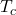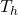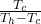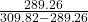A Carnot air conditioner takes energy from the thermal energy of a room at 61°F and transfers it as heat to the outdoors, which is at 98°F.

Question

A Carnot air conditioner takes energy from the thermal energy of a room at 61°F and transfers it as heat to the outdoors, which is at 98°F. For each joule of electric energy required to operate the air conditioner, how many joules are removed from the room?

in progress 0
6 months 2021-08-11T11:05:33+00:00 1 Answers 5 views 0

14.07 units of joules is removed for each joules of electricity used

Explanation:

The heated room temperature= 61 °F

The outside temperature= 98 °F

For conversion from fahrenheit to kelvin we use the equation

(32°F − 32) × 5/9 + 273.15= 289.26= 309.82

For air conditioning,

COP =COP == 14.07

This means that 14.07 units of joules is removed for each joules of electricity used